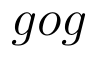Select Page

# Differential Equations CBSE Maths 12 Science MCQ Answers in English

Differential Equations CBSE Maths 12 Science MCQ Answers in English to enable students to get Answers in a narrative video format for the specific question.

Expert Teacher provides Differential Equations CBSE Maths 12 Science MCQ Answers through Video Answers in English language. This video solution will be useful for students to understand how to write an answer in exam in order to score more marks. This teacher uses a narrative style for a question from Differential Equations not only to explain the proper method of answering question, but deriving right answer too.

Please find the question below and view the Answer in a narrative video format.

Question:

## Similar Questions from CBSE, 12th Science, Maths, Differential Equations

Question 1 : If y(x) is a solution of the differential equationand y(0) = 1, then find the value of(View Answer Video)

Question 2 : Solve the differential equationgiven that y = 1 when x = 1. (View Answer Video)

Question 3 : Form the differential equation of equation y = a cos 2x + b sin 2x, where a and b are constant. (View Answer Video)

Question 5 : Find the particular solution of the differential equationgiven that y = 0, when x = 0. (View Answer Video)

### Application of Integrals

Question 1 : Find the area of the region bounded by the parabolaand y = | x |. (View Answer Video)

Question 2 : Find the area of the region. (View Answer Video)

Question 3 : Find the area between the curves y = x and(View Answer Video)

Question 4 : Using the method of integration, find the area of the triangular region whose vertices are (2, -2), (4, 3) and (1, 2). (View Answer Video)

Question 5 : Using integration, find the area bounded by the tangent to the curveat the point (2, 1) and the lines whose equations are x = 2y and x = 3y - 3. (View Answer Video)

### Relations and Functions

Question 1 : Functionsare defined respectively, by, find(View Answer Video)

Question 2 : A functiondefined as f(x) = 5x is, (View Answer Video)

Question 3 : Let A = {1, 2, 3}. Then, number of equivalence relations containing (1, 2) are, (View Answer Video)

Question 4 : Letbe defined as. Choose the correct answer. (View Answer Video)

Question 5 :  Letdefined as f(x) = 5 be a constant function. Then its range is (View Answer Video)

### Continuity and Differentiability

Question 1 : Differentiate the functionwith respect to x. (View Answer Video)

Question 2 : If x and y are connected parametrically by the equation, without eliminating the parameter, find. (View Answer Video)

Question 3 : Differentiate the function w.r.t.x. (View Answer Video)

Question 4 : Differentiate the functionwith respect to x. (View Answer Video)

Question 5 : Findfor the function. (View Answer Video)